# Does welding require math?

Welding is a vital process that brings different metal pieces together to create a robust structure.

It’s a technique used in various industries such as construction, automotive, and manufacturing. While welding requires technical skills, many people wonder whether math plays a role in this field.

The answer is yes. Math is an integral part of welding, and it’s crucial to understand its fundamentals.

Welders use mathematical concepts and formulas to ensure their work is precise and accurate. They calculate the length of metal pieces needed for a project, determine the exact angles required to join the metal, and apply algebra, geometry, and trigonometry principles to complete their tasks safely and efficiently.

In short, math is involved in every step of the welding process. In this blog post, we’ll explore why math is essential in welding and highlight some key mathematical concepts that welders use daily.

So, let’s dive into the world of welding and discover how math makes all the difference.

## Does Welding Require Math?

The short answer is yes – math is crucial to welding.

Welding requires precision and accuracy, and welders use math skills every day on the job. The types of math skills used in welding range from basic arithmetic to more advanced concepts such as geometry, trigonometry, and algebra.

For instance, when welding two pieces of metal together, a welder must calculate the correct length of the filler metal needed to make a strong bond between the two pieces. Welders also need to understand angles, shapes, and dimensions.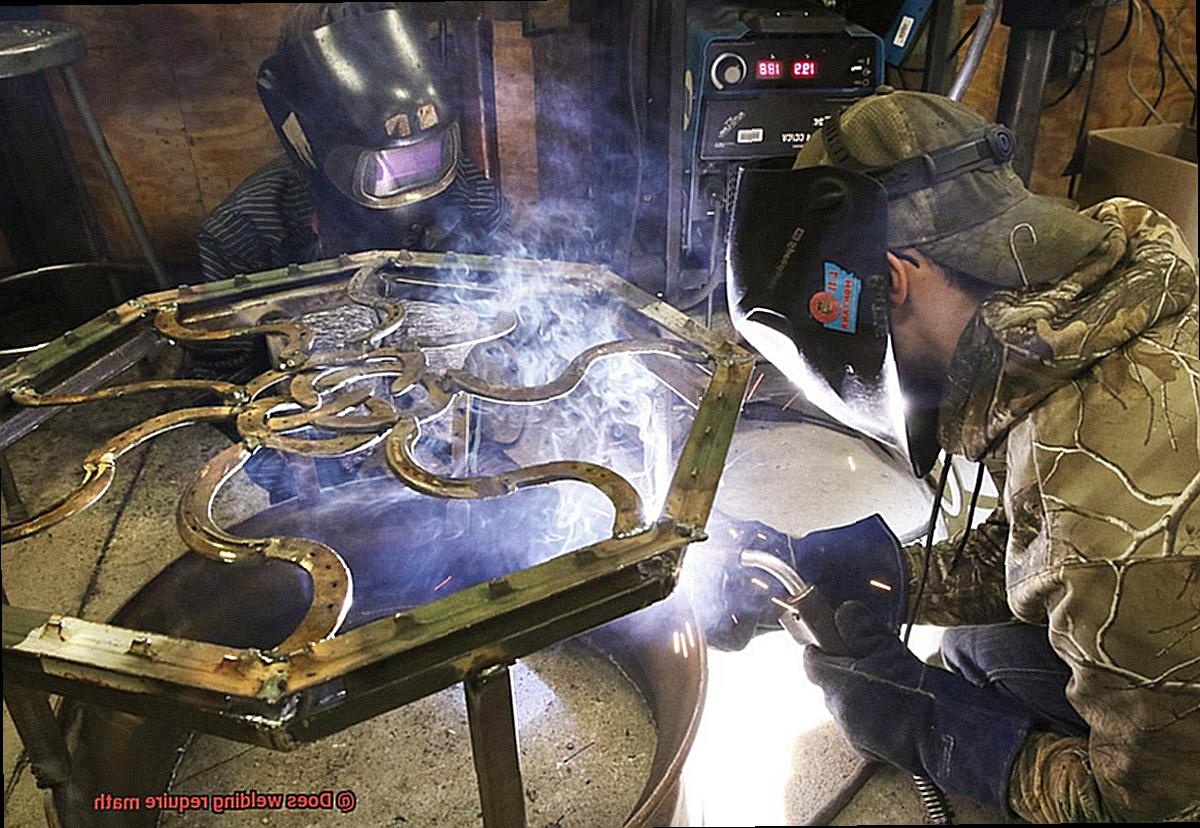They must be able to read blueprints and schematics accurately, which require a solid understanding of geometry and trigonometry. While some welding jobs may require more advanced math concepts such as algebra, it’s not typically necessary for most welding tasks.

However, having a strong foundation in math can make a welder’s job easier and more efficient. Moreover, welders working in aerospace or manufacturing may be required to work with more complex materials such as titanium or composites that require higher level math knowledge like trigonometry and geometry to calculate angles and dimensions accurately.

Welders must have a solid understanding of basic math concepts to ensure that their finished product meets specifications.

## Types of Math Skills Required for Welding

Welding is a craft that demands precision and accuracy – and that’s where math comes in.

Math skills are essential for welders to measure, calculate, and determine the correct technique for each project. In this post, we will explore the different types of math skills required for welding.

First and foremost, measurement skills are critical for welders. Using measuring tools such as rulers, calipers, and micrometers, they must measure accurately and precisely.

This skill is vital to ensure that welding projects meet the necessary specifications. Secondly, geometry is another math skill required for welding.

Welders need to understand geometric shapes and how they fit together to create a finished product. They must calculate angles, triangles, and other shapes to ensure that the weld is done correctly.

Additionally, algebra plays a significant role in welding. Welders use algebra to calculate the amount of material needed for a project or determine the proper welding technique.

Algebra is also used to calculate the amount of heat needed for a weld or to determine the correct amount of pressure for a specific type of weld. Lastly, understanding basic physics concepts is crucial for welders.

They must also understand the laws of motion and how they apply to welding.

## Math Skills Needed for Construction Welders

If you’re curious about the math skills needed for construction welders, look no further. As an expert in this field, I’ll walk you through the essential math skills you need to become a successful construction welder.

First and foremost, basic math concepts like addition, subtraction, multiplication, and division are fundamental for construction welders. These skills help you understand measurements and dimensions, which are crucial for reading and interpreting blueprints and sketches accurately.

But welding requires more than just the basics. Construction welders also need to master geometry, trigonometry, and algebra.

These skills play a crucial role in determining angles, calculating the length of welding material required for a job, and understanding the properties of different metals used in welding. However, constructing welding also demands excellent problem-solving abilities.

On the job, welders often face unexpected challenges that require them to think critically and come up with solutions quickly. Math skills are an integral part of this problem-solving process.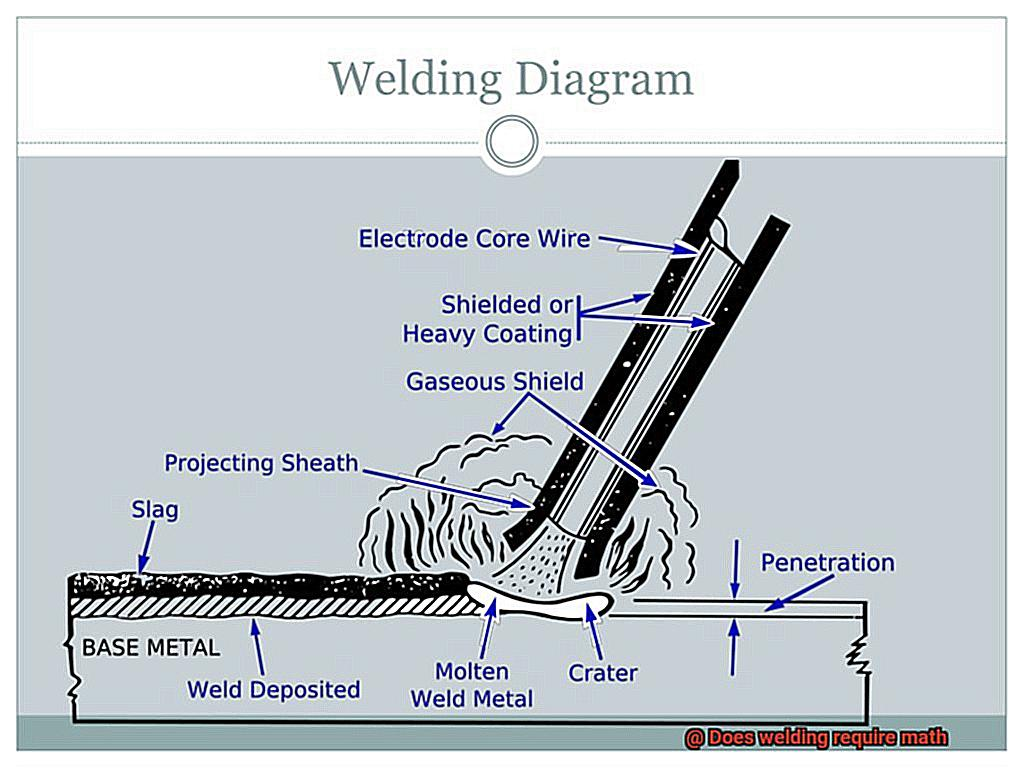Now, if you’re worried about mastering these math skills, don’t be. With training and practice, most welders can develop the necessary math skills to perform their job effectively.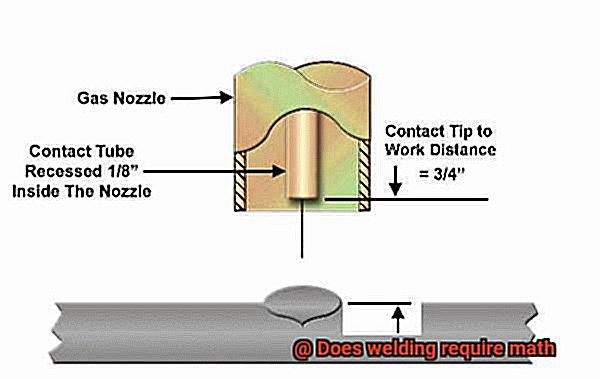It’s just like learning any dance routine; it may seem complicated at first, but with persistence and practice, it becomes second nature. In conclusion, math skills are vital for construction welders.

## Math Skills Needed for Aerospace and Manufacturing Welders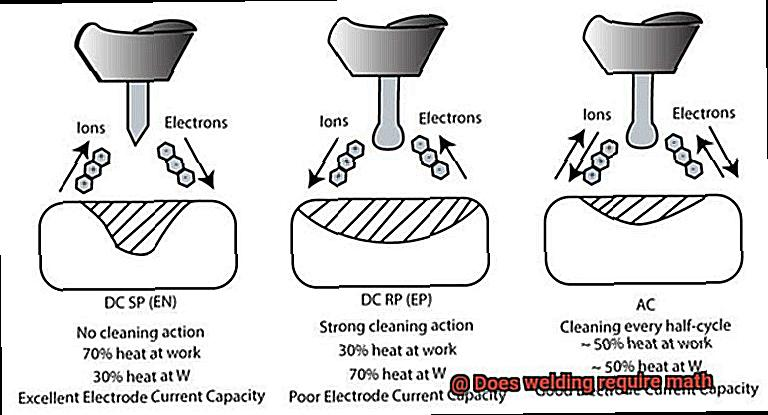As a welder in the aerospace industry, precision and accuracy are paramount. A small miscalculation can have serious consequences, which is why a strong understanding of trigonometry, geometry, and calculus is necessary to accurately measure and cut metal to the required specifications.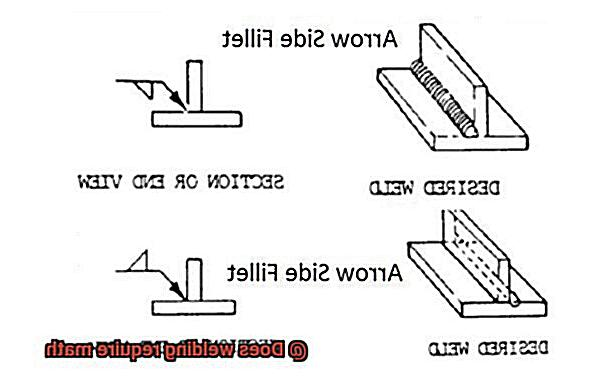It’s like performing a dance routine, where every step must be executed flawlessly to create a beautiful finished product. While manufacturing welding requires math skills at a slightly lower level than aerospace welding, they are still fundamental to success in this field.

Manufacturing welders need to be able to read blueprints and schematics with ease, which requires a basic understanding of geometry and algebra. They may also need to perform calculations to determine the amount of materials required for a project and their costs.

But don’t let math scare you away from these exciting fields. With practice and training, even those who struggle with math can become proficient welders.

It’s like learning a new dance routine; at first, the steps may be challenging, but with time and repetition, they become second nature. So if you’re considering a career in aerospace or manufacturing welding, get ready to embrace the power of math.

From measuring metal to calculating costs, these skills are crucial for success in these fields.

## Using Algebra in Welding

From calculating materials to determining angles and understanding the properties of different metals, algebra plays a significant role in welding.

Let’s start with calculating the amount of material needed for a project.

Welders must measure the length, width, and thickness of the metal they will be working with to determine the volume of material needed.

This calculation requires knowledge of basic algebraic equations such as volume = length x width x height. It’s like baking a cake – you need to know the right amount of each ingredient to make it perfectly.

Another crucial use of algebra in welding is determining angles. Welders must accurately calculate angles to ensure their welded joints are strong and secure.

They must use trigonometric functions like sine, cosine, and tangent to find the angle measurements. Imagine trying to build a tower without knowing the right angles – it would be impossible to create a stable structure.

In addition to these applications, algebra is also used in understanding the properties of different metals. Welders must know how various metals react under different conditions such as temperature and pressure.

Understanding these properties requires knowledge of algebraic equations like thermal expansion and electrical resistance. It’s like having a map that guides you through unfamiliar terrain.

Algebra is like the backbone of welding – it helps welders execute flawless moves and create perfect finished products. Without these mathematical skills, welders could potentially make mistakes that could compromise the strength and durability of their welded joints.

## Other Math Skills Used in Welding

Welding is not just a craft that relies on physical dexterity and skill.

It demands a high level of precision, which is why math plays an important role in ensuring that the finished product meets the required standards. As an expert in welding, I can attest to the significance of math skills in this field.

Geometry is crucial in welding, as it helps welders determine angles, shapes, and dimensions of the materials they are working with. The ability to read blueprints and understand technical drawings is a must, and this often requires knowledge of trigonometry and calculus.

By using these mathematical concepts, welders can ensure that their finished product conforms to precise specifications. Measurement is another vital aspect of welding that requires math skills.

Welders need to be proficient in using measuring tools such as rulers, tape measures, and protractors to ensure accurate cuts and welds. They also need to be able to convert between different units of measurement, such as inches to millimeters or feet to meters.

This means that welders need to have a good grasp of basic arithmetic and be able to perform calculations quickly and accurately. In addition, algebra is an essential skill for welders.

They need to calculate the amount of materials needed for a project based on the length, width, and thickness of metal sheets or pipes. This requires an understanding of basic algebra and the ability to solve equations.

A slight miscalculation in this area could lead to wasted materials or even compromise the safety and durability of the finished product. In conclusion, math skills are integral to welding.

GecHIpR8aoE” >

## Conclusion

To sum up, the answer to the question “Does welding require math?”

It is a resounding yes. Welding demands accuracy and precision, making math skills essential for welders to measure, calculate, and determine the correct technique for each project.

Welders utilize mathematical concepts and formulas to ensure their work is precise and accurate. From calculating the length of metal pieces needed for a project to determining exact angles required to join metals, welders apply arithmetic, geometry, trigonometry, and algebra principles to complete their tasks safely and efficiently.

The types of math skills used in welding vary from basic arithmetic to more advanced concepts such as geometry, trigonometry, and algebra. Welders must have a solid understanding of fundamental math concepts like addition, subtraction, multiplication, division as well as measurement skills.

Additionally, they need mastery in geometry, trigonometry and algebra. Furthermore, welders working in aerospace or manufacturing may be required to work with more complex materials such as titanium or composites that demand higher level math knowledge like trigonometry and geometry to calculate angles and dimensions accurately.

With practice and training even those who struggle with math can become proficient welders.